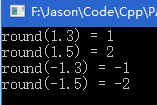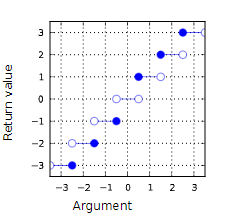# C / C + + rounding function: round function

: : : : : : : : : : : : : : :

``````#include<iostream>
#include<cmath>
using namespace std;

int main(){
cout<<"round(1.3) = "<<round(1.3)<<endl
<<"round(1.5) = "<<round(1.5)<<endl
<<"round(-1.3) = "<<round(-1.3)<<endl
<<"round(-1.5) = "<<round(-1.5)<<endl;
return 0;
}
``````this function takes the form of

``````double round(double d);
``````

> > > > > > > > > > cmath>